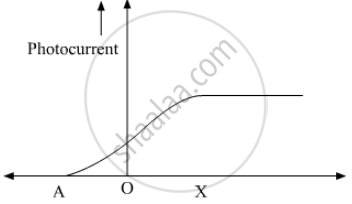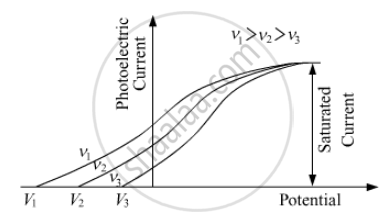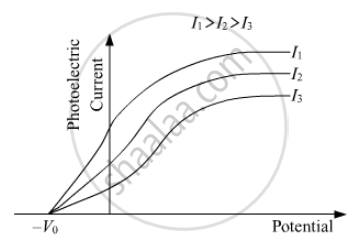Department of Pre-University Education, KarnatakaPUC Karnataka Science Class 12

# The Following Graph Shows the Variation of Photocurrent for a Photosensitive Metal - Physics

The following graph shows the variation of photocurrent for a photosensitive metal :(a) Identify the variable X on the horizontal axis.

(b) What does the point A on the horizontal axis represent?

(c) Draw this graph for three different values of frequencies of incident radiation v1, v2 and v3 (v1 > v2 > v3) for same intensity.

(d) Draw this graph for three different values of intensities of incident radiation I1, I2 and I3 (I1 > I2 > I3) having same frequency.

#### Solution

(a) Variable X is the accelerating potential applied across the photosensitive material.

(b) A represents the stopping potential for the given photosensitive metal. Stopping potential is the minimum negative potential V0 applied across the photosensitive material at which the photoelectric current becomes zero.

(c) Graph for three different values of frequencies of incident radiation v1, v2 and v3 (v1 > v2 > v3) for same intensity(d) Graph for three different values of intensities of incident radiation I1, I2 and I3 (I1 > I2 > I3) having same frequencyConcept: Experimental Study of Photoelectric Effect
Is there an error in this question or solution?